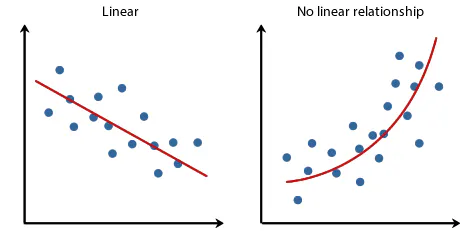Linear Regression and its Mathematical implementationby@afroz-chakure

# Linear Regression and its Mathematical implementation

August 9th 2019
2mLinear Regression is a predictive statistical approach for modelling relationship between a dependent variable with a given set of independent variables. The linear equation assigns one scale factor to each input value or column, called a coefficient and represented by the capital Greek letter Beta (B) For more than one independent variable, the process is called as multiple linear regression. The General equation for a Multiple linear regression with p - independent variables looks like this: B0 + B1*x, where B0 represents the intercept or the bias coefficient.### @afroz-chakure

Afroz Chakure